# Difference between revisions of "Carnahan-Starling equation of state"

The Carnahan-Starling equation of state is an approximate (but quite good) equation of state for the fluid phase of the hard sphere model in three dimensions. It is given by (Ref  Eqn. 10).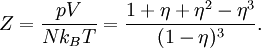$Z = \frac{ p V}{N k_B T} = \frac{ 1 + \eta + \eta^2 - \eta^3 }{(1-\eta)^3 }.$

where:

•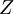$Z$ is the compressibility factor
•$p$ is the pressure
•$V$ is the volume
•$N$ is the number of particles
•$k_B$ is the Boltzmann constant
•$T$ is the absolute temperature
•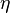$\eta$ is the packing fraction: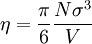$\eta = \frac{ \pi }{6} \frac{ N \sigma^3 }{V}$
•$\sigma$ is the hard sphere diameter.

The Carnahan-Starling equation of state is not applicable for packing fractions greater than 0.55 .

## Virial expansion

It is interesting to compare the virial coefficients of the Carnahan-Starling equation of state (Eq. 7 of ) with the hard sphere virial coefficients in three dimensions (exact up to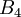$B_4$, and those of Clisby and McCoy ):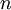$n$ Clisby and McCoy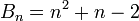$B_n=n^2+n-2$ 2 4 4 3 10 10 4 18.3647684 18 5 28.224512 28 6 39.8151475 40 7 53.3444198 54 8 68.5375488 70 9 85.8128384 88 10 105.775104 108

## Thermodynamic expressions

From the Carnahan-Starling equation for the fluid phase the following thermodynamic expressions can be derived (Ref  Eqs. 2.6, 2.7 and 2.8)

Pressure (compressibility):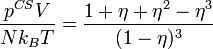$\frac{p^{CS}V}{N k_B T } = \frac{1+ \eta + \eta^2 - \eta^3}{(1-\eta)^3}$

Configurational chemical potential: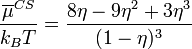$\frac{ \overline{\mu }^{CS}}{k_B T} = \frac{8\eta -9 \eta^2 + 3\eta^3}{(1-\eta)^3}$

Isothermal compressibility: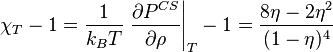$\chi_T -1 = \frac{1}{k_BT} \left.\frac{\partial P^{CS}}{\partial \rho}\right\vert_{T} -1 = \frac{8\eta -2 \eta^2 }{(1-\eta)^4}$

where$\eta$ is the packing fraction.

Configurational Helmholtz energy function: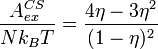$\frac{ A_{ex}^{CS}}{N k_B T} = \frac{4 \eta - 3 \eta^2 }{(1-\eta)^2}$

## The 'Percus-Yevick' derivation

It is interesting to note (Ref  Eq. 6) that one can arrive at the Carnahan-Starling equation of state by adding two thirds of the exact solution of the Percus Yevick integral equation for hard spheres via the compressibility route, to one third via the pressure route, i.e.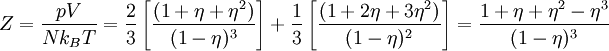$Z = \frac{ p V}{N k_B T} = \frac{2}{3} \left[ \frac{(1+\eta+\eta^2)}{(1-\eta)^3} \right] + \frac{1}{3} \left[ \frac{(1+2\eta+3\eta^2)}{(1-\eta)^2} \right] = \frac{ 1 + \eta + \eta^2 - \eta^3 }{(1-\eta)^3 }$

The reason for this seems to be a slight mystery (see discussion in Ref.  ).

## Kolafa correction

Jiri Kolafa produced a slight correction to the C-S EOS which results in improved accuracy :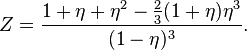$Z = \frac{ 1 + \eta + \eta^2 - \frac{2}{3}(1+\eta) \eta^3 }{(1-\eta)^3 }.$